# Chapter 11 Linear Regression and Correlation Linear Regression

• Slides: 23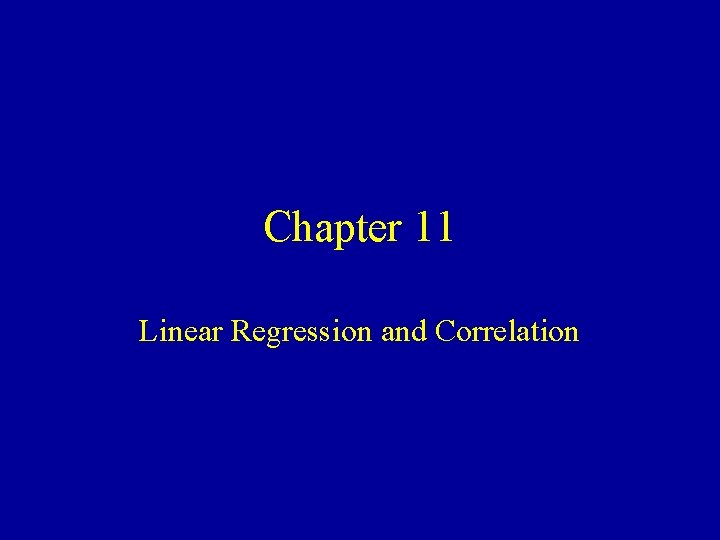Chapter 11 Linear Regression and Correlation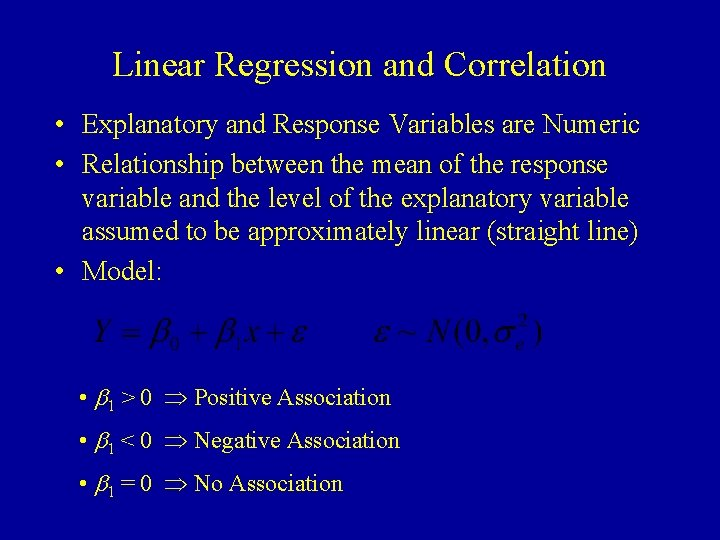Linear Regression and Correlation • Explanatory and Response Variables are Numeric • Relationship between the mean of the response variable and the level of the explanatory variable assumed to be approximately linear (straight line) • Model: • b 1 > 0 Positive Association • b 1 < 0 Negative Association • b 1 = 0 No Association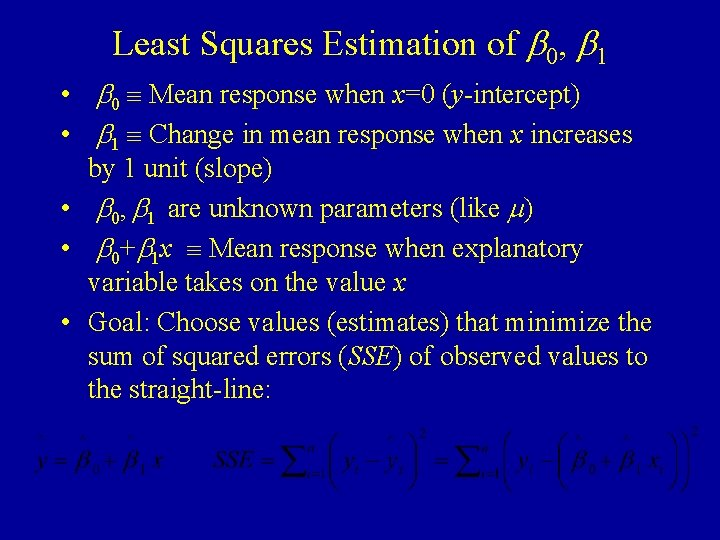Least Squares Estimation of b 0, b 1 • b 0 Mean response when x=0 (y-intercept) • b 1 Change in mean response when x increases by 1 unit (slope) • b 0, b 1 are unknown parameters (like m) • b 0+b 1 x Mean response when explanatory variable takes on the value x • Goal: Choose values (estimates) that minimize the sum of squared errors (SSE) of observed values to the straight-line: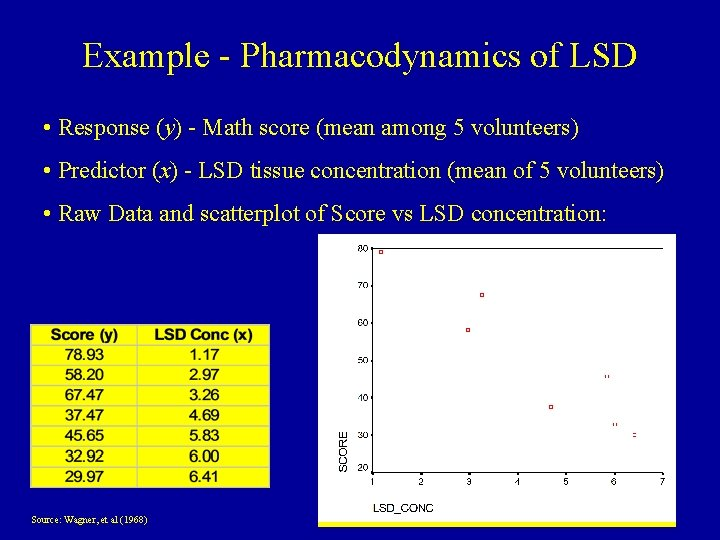Example - Pharmacodynamics of LSD • Response (y) - Math score (mean among 5 volunteers) • Predictor (x) - LSD tissue concentration (mean of 5 volunteers) • Raw Data and scatterplot of Score vs LSD concentration: Source: Wagner, et al (1968)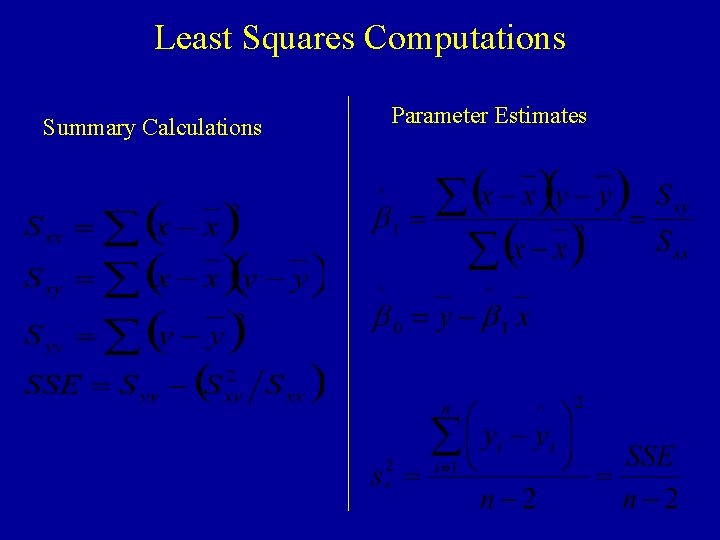Least Squares Computations Summary Calculations Parameter Estimates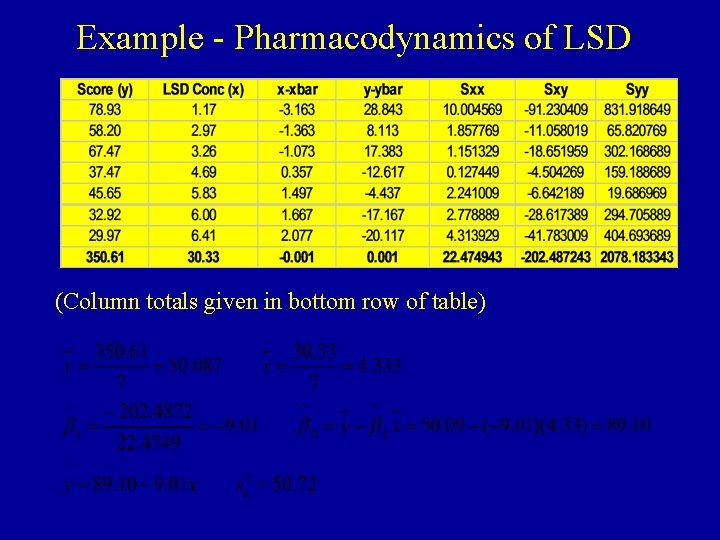Example - Pharmacodynamics of LSD (Column totals given in bottom row of table)SPSS Output and Plot of Equation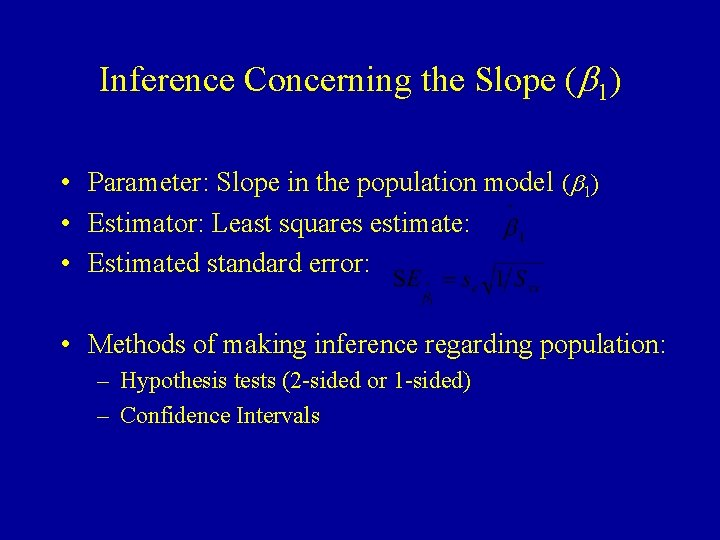Inference Concerning the Slope (b 1) • Parameter: Slope in the population model (b 1) • Estimator: Least squares estimate: • Estimated standard error: • Methods of making inference regarding population: – Hypothesis tests (2 -sided or 1 -sided) – Confidence IntervalsHypothesis Test for b 1 • 2 -Sided Test – H 0: b 1 = 0 – H A: b 1 0 • 1 -sided Test – H 0: b 1 = 0 – HA+: b 1 > 0 or – H A -: b 1 < 0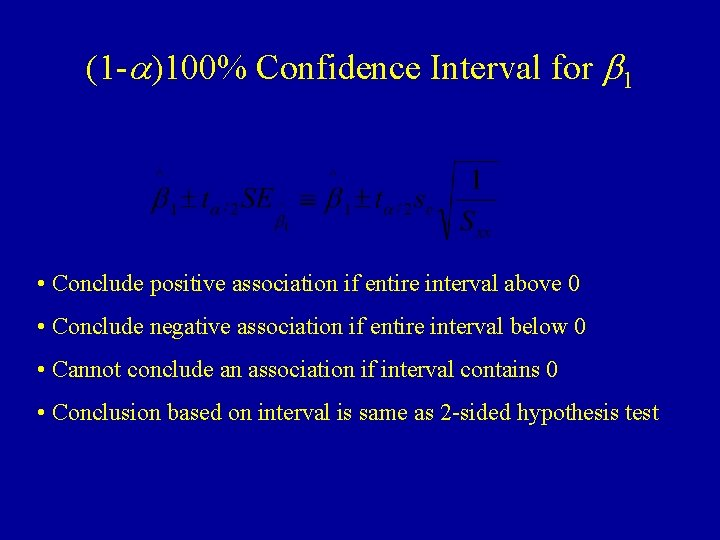(1 -a)100% Confidence Interval for b 1 • Conclude positive association if entire interval above 0 • Conclude negative association if entire interval below 0 • Cannot conclude an association if interval contains 0 • Conclusion based on interval is same as 2 -sided hypothesis testExample - Pharmacodynamics of LSD • Testing H 0: b 1 = 0 vs HA: b 1 0 • 95% Confidence Interval for b 1 :Confidence Interval for Mean When x=x* • Mean Response at a specific level x* is • Estimated Mean response and standard error (replacing unknown b 0 and b 1 with estimates): • Confidence Interval for Mean Response: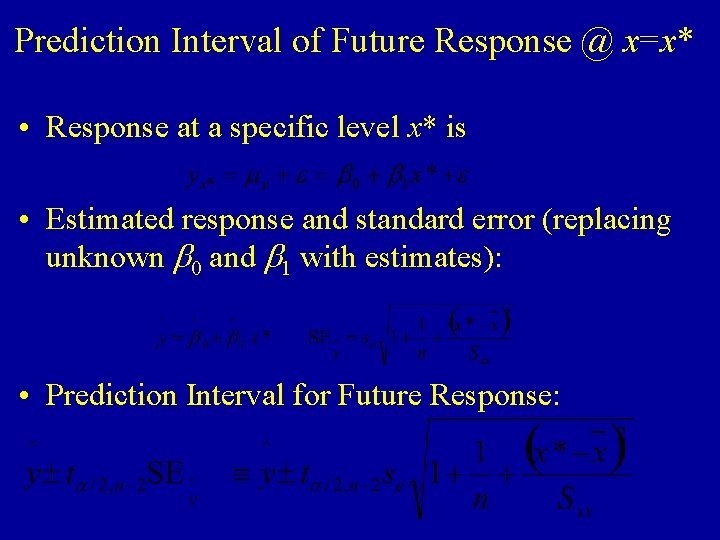Prediction Interval of Future Response @ x=x* • Response at a specific level x* is • Estimated response and standard error (replacing unknown b 0 and b 1 with estimates): • Prediction Interval for Future Response: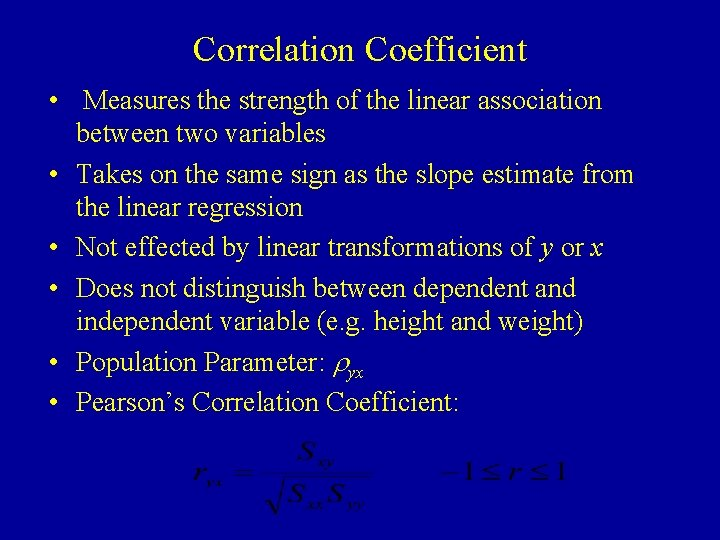Correlation Coefficient • Measures the strength of the linear association between two variables • Takes on the same sign as the slope estimate from the linear regression • Not effected by linear transformations of y or x • Does not distinguish between dependent and independent variable (e. g. height and weight) • Population Parameter: ryx • Pearson’s Correlation Coefficient: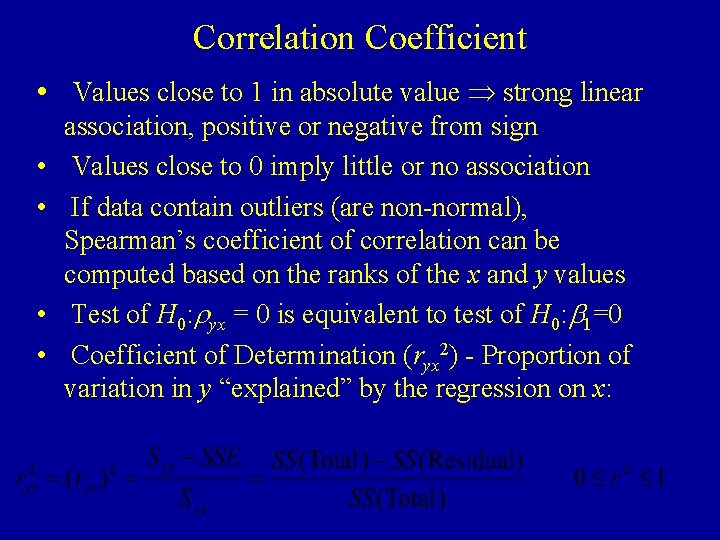Correlation Coefficient • Values close to 1 in absolute value strong linear • • association, positive or negative from sign Values close to 0 imply little or no association If data contain outliers (are non-normal), Spearman’s coefficient of correlation can be computed based on the ranks of the x and y values Test of H 0: ryx = 0 is equivalent to test of H 0: b 1=0 Coefficient of Determination (ryx 2) - Proportion of variation in y “explained” by the regression on x:Example - Pharmacodynamics of LSD Syy SSEExample - SPSS Output Pearson’s and Spearman’s MeasuresHypothesis Test for ryx • 2 -Sided Test – H 0: ryx = 0 – HA: ryx 0 • 1 -sided Test – H 0: ryx = 0 – HA+: ryx > 0 or – HA-: ryx < 0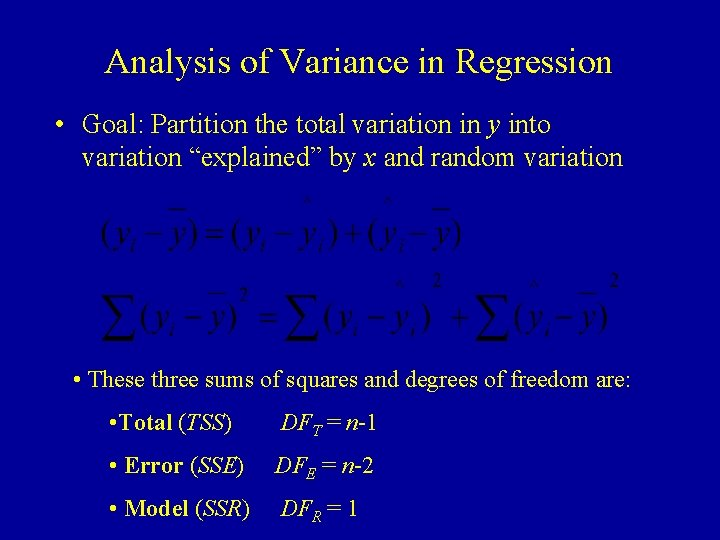Analysis of Variance in Regression • Goal: Partition the total variation in y into variation “explained” by x and random variation • These three sums of squares and degrees of freedom are: • Total (TSS) DFT = n-1 • Error (SSE) DFE = n-2 • Model (SSR) DFR = 1Analysis of Variance for Regression • Analysis of Variance - F-test • H 0: b 1 = 0 HA: b 1 0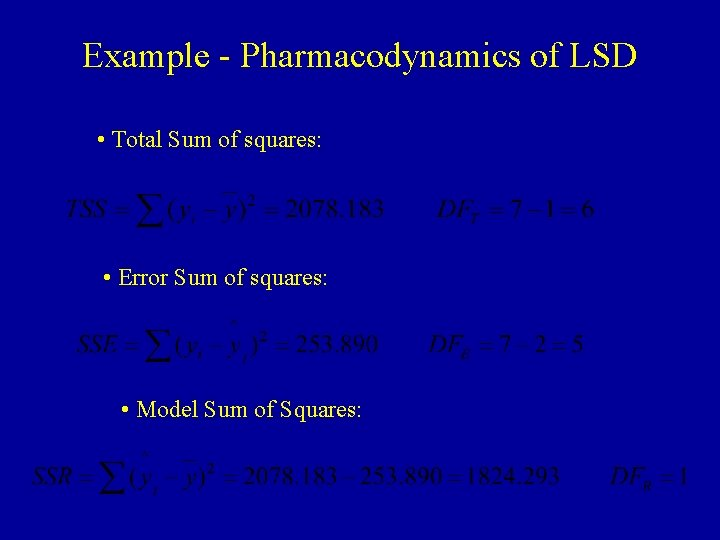Example - Pharmacodynamics of LSD • Total Sum of squares: • Error Sum of squares: • Model Sum of Squares:Example - Pharmacodynamics of LSD • Analysis of Variance - F-test • H 0: b 1 = 0 HA: b 1 0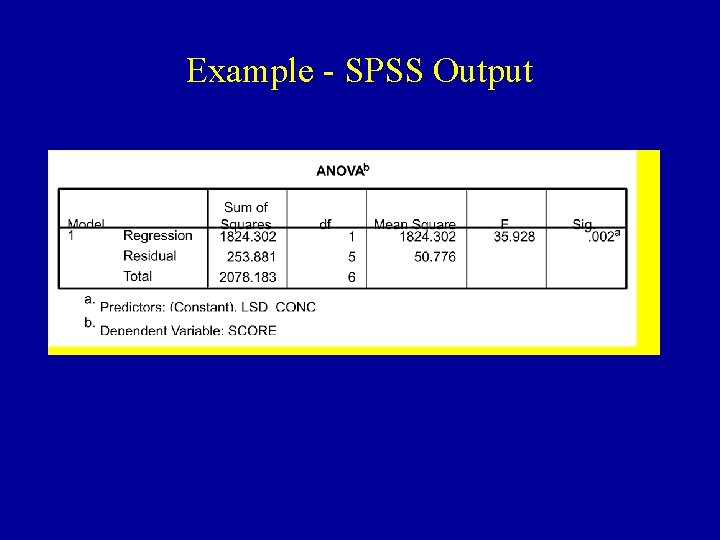Example - SPSS Output